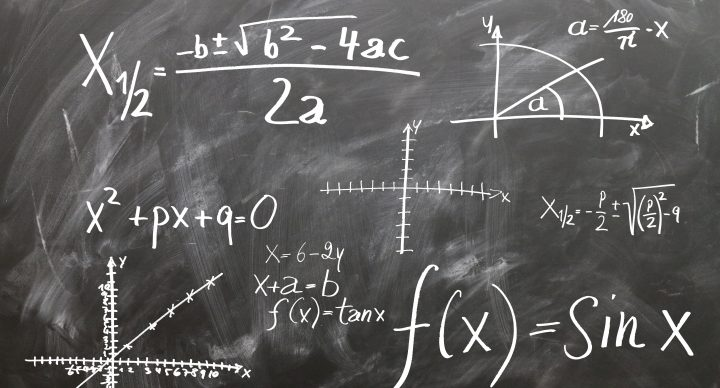# Algebra I (Advanced Algebra I)### Course Overview

Algebra I, taught by Mark Rogers, presents algebraic concepts on a high school level. Students learn about algebraic expressions and equations. They are introduced to various functions, including linear, exponential, quadratic, inverse, and square root functions. This course is recommended for high school and gifted middle school students who have successfully completed a Pre-Algebra course.
Course topics include:
• Algebraic Expressions and Equations
• Proportions, Inequalities, and Absolute Values
• Introduction to Functions
• Linear Functions and Systems
• Exponential Properties and Functions
• Factoring
• Quadratic, Inverse, and Square Root Functions
• Statistical Analysis
Acellus Algebra I is taught by Acellus Instructor Mark Rogers.

### Sample Lesson - Algebraic ExpressionThis course was developed by the International Academy of Science. Learn More